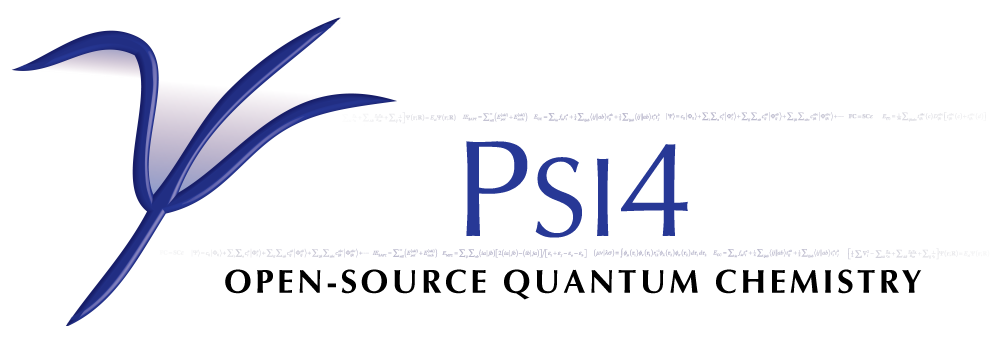# PSI Variables by Alpha¶

Note

Lowercase letters in PSI variable names represent portions of the variable name that vary by root number, calculation order, etc. See text for fuller description.

(T) CORRECTION ENERGY

The coupled-cluster perturbative triples correction [H].

A-(T) CORRECTION ENERGY

The coupled-cluster asymmetric perturbative triples correction [H].

MP4(T) CORRECTION ENERGY

The MP4 triples component [H]. Quantity is second right-hand term in Eq. (2).

AAA (T) CORRECTION ENERGY
AAB (T) CORRECTION ENERGY
ABB (T) CORRECTION ENERGY
BBB (T) CORRECTION ENERGY

Spin components of the UHF-based coupled-cluster perturbative triples correction [H].

ACPF DIPOLE X
ACPF DIPOLE Y
ACPF DIPOLE Z

The three components of the dipole [Debye] for the averaged coupled-pair functional level of theory.

ACPF QUADRUPOLE XX
ACPF QUADRUPOLE XY
ACPF QUADRUPOLE XZ
ACPF QUADRUPOLE YY
ACPF QUADRUPOLE YZ
ACPF QUADRUPOLE ZZ

The six components of the quadrupole [Debye Ang] for the averaged coupled-pair functional level of theory.

ACPF TOTAL ENERGY
ACPF CORRELATION ENERGY

The total electronic energy [H] and correlation energy component [H] for the averaged coupled-pair functional level of theory.

AQCC DIPOLE X
AQCC DIPOLE Y
AQCC DIPOLE Z

The three components of the dipole [Debye] for the averaged quadratic coupled-cluster level of theory.

AQCC QUADRUPOLE XX
AQCC QUADRUPOLE XY
AQCC QUADRUPOLE XZ
AQCC QUADRUPOLE YY
AQCC QUADRUPOLE YZ
AQCC QUADRUPOLE ZZ

The six components of the quadrupole [Debye Ang] for the averaged quadratic coupled-cluster level of theory.

AQCC TOTAL ENERGY
AQCC CORRELATION ENERGY

The total electronic energy [H] and correlation energy component [H] for the averaged quadratic coupled-cluster level of theory.

BRUECKNER CONVERGED

Value 1 (0) when the Brueckner orbitals have (have not) converged.

CBS TOTAL ENERGY
CBS CORRELATION ENERGY
CBS REFERENCE ENERGY

The total electronic energy [H] and its breakdown into reference total energy [H] and correlation correction components [H] for the compound method requested through cbs().

CC ROOT n TOTAL ENERGY

The total electronic energy [H] for the requested coupled cluster level of theory and root n (numbering starts at GS = 0).

CC TOTAL ENERGY
CC CORRELATION ENERGY
CC2 TOTAL ENERGY
CC2 CORRELATION ENERGY
CC3 TOTAL ENERGY
CC3 CORRELATION ENERGY
CC4 TOTAL ENERGY
CC4 CORRELATION ENERGY
CCnn TOTAL ENERGY
CCnn CORRELATION ENERGY

The total electronic energy [H] and correlation energy component [H] for the requested approximate coupled-cluster (CC2, CC3, up to CCnn) level of theory.

CC DIPOLE X
CC DIPOLE Y
CC DIPOLE Z

The three components of the dipole [Debye] for the requested coupled cluster level of theory and root.

CC QUADRUPOLE XX
CC QUADRUPOLE XY
CC QUADRUPOLE XZ
CC QUADRUPOLE YY
CC QUADRUPOLE YZ
CC QUADRUPOLE ZZ

The six components of the quadrupole [Debye Ang] for the requested coupled cluster level of theory and root.

CCSD TOTAL ENERGY
CCSD CORRELATION ENERGY
CCSDT TOTAL ENERGY
CCSDT CORRELATION ENERGY
CCSDTQ TOTAL ENERGY
CCSDTQ CORRELATION ENERGY
CCn TOTAL ENERGY
CCn CORRELATION ENERGY

The total electronic energy [H] and correlation energy component [H] for the requested full coupled-cluster (CCSD, CCSDT, up to CCn) level of theory.

CCSD(T) TOTAL ENERGY
CCSD(T) CORRELATION ENERGY
A-CCSD(T) TOTAL ENERGY
A-CCSD(T) CORRELATION ENERGY
CCSDT(Q) TOTAL ENERGY
CCSDT(Q) CORRELATION ENERGY
CC(n-1)(n) TOTAL ENERGY
CC(n-1)(n) CORRELATION ENERGY

The total electronic energy [H] and correlation energy component [H] for the perturbatively corrected coupled-cluster (CCSD(T), a-CCSD(T), CCSDT(Q), up to CC(n-1)(n) level of theory.

CCSDT-1a TOTAL ENERGY
CCSDT-1a CORRELATION ENERGY
CCSDTQ-1a TOTAL ENERGY
CCSDTQ-1a CORRELATION ENERGY
CCn-1a TOTAL ENERGY
CCn-1a CORRELATION ENERGY

The total electronic energy [H] and correlation energy component [H] for the approximate coupled-cluster (CCSD(T)-1a, CCSDT(Q)-1a, up to CCn-1a) level of theory.

CCSDT-1b TOTAL ENERGY
CCSDT-1b CORRELATION ENERGY
CCSDTQ-1b TOTAL ENERGY
CCSDTQ-1b CORRELATION ENERGY
CCn-1b TOTAL ENERGY
CCn-1b CORRELATION ENERGY

The total electronic energy [H] and correlation energy component [H] for the approximate coupled-cluster (CCSD(T)-1b, CCSDT(Q)-1b, up to CCn-1b) level of theory.

CCSDT-3 TOTAL ENERGY
CCSDT-3 CORRELATION ENERGY
CCSDTQ-3 TOTAL ENERGY
CCSDTQ-3 CORRELATION ENERGY
CCn-3 TOTAL ENERGY
CCn-3 CORRELATION ENERGY

The total electronic energy [H] and correlation energy component [H] for the approximate coupled-cluster (CCSD(T)-3, CCSDT(Q)-3, up to CCn-3) level of theory.

CCSD(T)_L TOTAL ENERGY
CCSD(T)_L CORRELATION ENERGY
CCSDT(Q)_L TOTAL ENERGY
CCSDT(Q)_L CORRELATION ENERGY
CC(n-1)(n)_L TOTAL ENERGY
CC(n-1)(n)_L CORRELATION ENERGY

The total electronic energy [H] and correlation energy component [H] for the approximate coupled-cluster (CCSD(T)_L, CCSDT(Q)_L, up to CC(n-1)(n)L level of theory.

CEPA(0) DIPOLE X
CEPA(0) DIPOLE Y
CEPA(0) DIPOLE Z

The three components of the dipole [Debye] for the coupled electron pair approximation variant 0 level of theory.

CEPA(0) QUADRUPOLE XX
CEPA(0) QUADRUPOLE XY
CEPA(0) QUADRUPOLE XZ
CEPA(0) QUADRUPOLE YY
CEPA(0) QUADRUPOLE YZ
CEPA(0) QUADRUPOLE ZZ

The six components of the quadrupole [Debye Ang] for the coupled electron pair approximation variant 0 level of theory.

CEPA(0) TOTAL ENERGY
CEPA(0) CORRELATION ENERGY
CEPA(1) TOTAL ENERGY
CEPA(1) CORRELATION ENERGY
CEPA(2) TOTAL ENERGY
CEPA(2) CORRELATION ENERGY
CEPA(3) TOTAL ENERGY
CEPA(3) CORRELATION ENERGY

The total electronic energy [H] and correlation energy component [H] for the requested variant of coupled electron pair approximation level of theory.

CFOUR ERROR CODE

The non-zero return value from a Cfour execution.

CI DIPOLE X
CI DIPOLE Y
CI DIPOLE Z

The three components of the dipole [Debye] for the requested configuration interaction level of theory and root.

CI QUADRUPOLE XX
CI QUADRUPOLE XY
CI QUADRUPOLE XZ
CI QUADRUPOLE YY
CI QUADRUPOLE YZ
CI QUADRUPOLE ZZ

The six components of the quadrupole [Debye Ang] for the requested configuration interaction level of theory and root.

CI ROOT n -> ROOT m DIPOLE X
CI ROOT n -> ROOT m DIPOLE Y
CI ROOT n -> ROOT m DIPOLE Z

The three components of the transition dipole [Debye] between roots n and m for the requested configuration interaction level of theory.

CI ROOT n -> ROOT m QUADRUPOLE XX
CI ROOT n -> ROOT m QUADRUPOLE XY
CI ROOT n -> ROOT m QUADRUPOLE XZ
CI ROOT n -> ROOT m QUADRUPOLE YY
CI ROOT n -> ROOT m QUADRUPOLE YZ
CI ROOT n -> ROOT m QUADRUPOLE ZZ

The three components of the transition quadrupole [Debye Ang] between roots n and m for the requested configuration interaction level of theory.

CI ROOT n DIPOLE X
CI ROOT n DIPOLE Y
CI ROOT n DIPOLE Z

The three components of the dipole [Debye] for the requested configuration interaction level of theory and root n.

CI ROOT n QUADRUPOLE XX
CI ROOT n QUADRUPOLE XY
CI ROOT n QUADRUPOLE XZ
CI ROOT n QUADRUPOLE YY
CI ROOT n QUADRUPOLE YZ
CI ROOT n QUADRUPOLE ZZ

The six components of the quadrupole [Debye Ang] for the requested configuration interaction level of theory and root n.

CI ROOT n TOTAL ENERGY
CI ROOT n CORRELATION ENERGY

The total electronic energy [H] and correlation energy component [H] for the requested configuration interaction level of theory and root n (numbering starts at 1).

CI STATE-AVERAGED TOTAL ENERGY
CI STATE-AVERAGED CORRELATION ENERGY

The total electronic energy [H] and correlation energy component [H] for state-averaged CI/CASSCF levels of theory.

CI TOTAL ENERGY
CI CORRELATION ENERGY

The total electronic energy [H] and correlation energy component [H] for the requested configuration interaction level of theory and root.

CISD DIPOLE X
CISD DIPOLE Y
CISD DIPOLE Z

The three components of the dipole [Debye] for the configuration interaction singles and doubles level of theory and root.

CISD QUADRUPOLE XX
CISD QUADRUPOLE XY
CISD QUADRUPOLE XZ
CISD QUADRUPOLE YY
CISD QUADRUPOLE YZ
CISD QUADRUPOLE ZZ

The six components of the quadrupole [Debye Ang] for the configuration interaction singles and doubles level of theory and root.

CISD TOTAL ENERGY
CISD CORRELATION ENERGY
CISDT TOTAL ENERGY
CISDT CORRELATION ENERGY
CISDTQ CORRELATION ENERGY
CISDTQ TOTAL ENERGY
CIn CORRELATION ENERGY
CIn TOTAL ENERGY

The total electronic energy [H] and correlation energy component [H] for the labeled configuration interaction level of theory and root. n is CI order for n > 4.

CP-CORRECTED 2-BODY INTERACTION ENERGY

The interaction energy [H] considering only two-body interactions, computed with counterpoise correction. Related variable UNCP-CORRECTED 2-BODY INTERACTION ENERGY.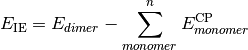CURRENT CORRELATION ENERGY

The correlation energy [H] corresponding to the CURRENT ENERGY variable.

CURRENT ENERGY

The total electronic energy [H] of the most recent stage of a calculation (frequently overwritten). This is the quantity tracked by the geometry optimizer.

CURRENT REFERENCE ENERGY

The total electronic energy [H] of the reference stage corresponding to the CURRENT ENERGY variable.

db_name DATABASE MEAN ABSOLUTE DEVIATION

The mean absolute deviation [kcal mol-1] of the requested method name from the stored reference values for the requested reactions in database db_name. If no reference is available, this will be a large and nonsensical value.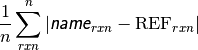db_name DATABASE MEAN SIGNED DEVIATION

The mean deviation [kcal mol-1] of the requested method name from the stored reference values for the requested reactions in database db_name. If no reference is available, this will be a large and nonsensical value.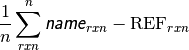db_name DATABASE ROOT-MEAN-SQUARE SIGNED DEVIATION

The rms deviation [kcal mol-1] of the requested method name from the stored reference values for the requested reactions in database db_name. If no reference is available, this will be a large and nonsensical value.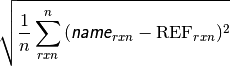DFT FUNCTIONAL TOTAL ENERGY

The total electronic energy [H] for the underlying functional of the requested DFT method, without any dispersion correction; the first four terms in Eq. (4) or (1). Quantity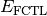in Eqs. (4) and (1). Unless the method includes a dispersion correction, this quantity is equal to SCF TOTAL ENERGY.

DFT TOTAL ENERGY

The total electronic energy [H] for the requested DFT method,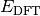in Eq. (1).

(1)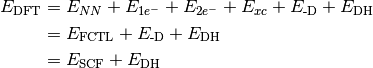Unless the method is a DFT double-hybrid, this quantity is equal to SCF TOTAL ENERGY. If the method is neither a double-hybrid, nor dispersion corrected, this quantity is equal to DFT FUNCTIONAL TOTAL ENERGY.

DFT XC ENERGY

The functional energy contribution [H] to the total SCF energy (DFT only). Quantity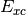in Eqs. (4) and (1).

DISPERSION CORRECTION ENERGY

The dispersion correction [H] appended to an underlying functional when a DFT-D method is requested. Quantity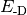in Eqs. (4) and (1).

DOUBLE-HYBRID CORRECTION ENERGY

The scaled MP2 correlation energy correction [H] appended to an underlying functional when a DH-DFT method is requested. Quantity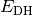in Eq. (1).

FCI TOTAL ENERGY
FCI CORRELATION ENERGY

The total electronic energy [H] and correlation energy component [H] for the full configuration interaction level of theory.

HF TOTAL ENERGY

The total electronic energy [H] for the Hartree–Fock method, without any dispersion correction; the first three (or four, since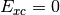) terms in Eq. (4). Quantity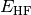in Eq. (4).

LCC2 (+LMP2) TOTAL ENERGY

The total electronic energy [H] for the local CC2 level of theory.

LCCSD (+LMP2) TOTAL ENERGY

The total electronic energy [H] for the local CCSD level of theory.

MP2 TOTAL ENERGY
MP2 CORRELATION ENERGY

The total electronic energy [H] and correlation energy component [H] for the MP2 level of theory.

MP2.5 TOTAL ENERGY
MP2.5 CORRELATION ENERGY

The total electronic energy [H] and correlation energy component [H] for the MP2.5 level of theory.

MP3 TOTAL ENERGY
MP3 CORRELATION ENERGY

The total electronic energy [H] and correlation energy component [H] for the MP3 level of theory.

MP4(SDQ) TOTAL ENERGY
MP4(SDQ) CORRELATION ENERGY

The total electronic energy [H] and correlation energy component [H] for the MP4 singles, doubles, quadruples level of theory. Quantity MP4(SDQ) CORRELATION ENERGY is first right-hand term in Eq. (2).

MP4 TOTAL ENERGY
MP4 CORRELATION ENERGY
MP4(SDTQ) TOTAL ENERGY
MP4(SDTQ) CORRELATION ENERGY

The total electronic energy [H] and correlation energy component [H] for the full MP4 level of theory. Quantity MP4 CORRELATION ENERGY / MP4(SDTQ) CORRELATION ENERGY is left-hand term in Eq. (2).

(2)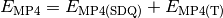MPn TOTAL ENERGY
MPn CORRELATION ENERGY

The total electronic energy [H] and correlation energy component [H] for the labeled Möller–Plesset perturbation theory level. n is MP perturbation order.

NUCLEAR REPULSION ENERGY

The nuclear repulsion energy contribution [H] to the total SCF energy. Quantity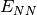in Eq. (4).

(3)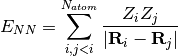OCEPA(0) TOTAL ENERGY
OCEPA(0) CORRELATION ENERGY

The total electronic energy [H] and correlation energy component [H] for the orbital-optimized CEPA(0) level of theory.

OMP2 TOTAL ENERGY
OMP2 CORRELATION ENERGY

The total electronic energy [H] and correlation energy component [H] for the orbital-optimized MP2 level of theory.

OMP3 TOTAL ENERGY
OMP3 CORRELATION ENERGY

The total electronic energy [H] and correlation energy component [H] for the orbital-optimized MP3 level of theory.

ONE-ELECTRON ENERGY

The one-electron energy contribution [H] to the total SCF energy. Quantity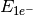in Eq. (4).

QCISD TOTAL ENERGY
QCISD CORRELATION ENERGY

The total electronic energy [H] and correlation energy component [H] for the quadratic configuration interaction singles and doubles level of theory.

QCISD(T) TOTAL ENERGY
QCISD(T) CORRELATION ENERGY

The total electronic energy [H] and correlation energy component [H] for the quadratic configuration interaction singles and doubles with perturbative triples correction level of theory.

SAPT DISP ENERGY
SAPT ELST ENERGY
SAPT EXCH ENERGY
SAPT IND ENERGY

Respectively, the dispersion, electrostatics, exchange, and induction components of the total electronic interaction energy [H] for the the requested SAPT level of theory. The sum of these four components yields SAPT TOTAL ENERGY.

SAPT TOTAL ENERGY

The total electronic interaction energy [H] for the requested SAPT level of theory.

SAPT0 TOTAL ENERGY
SSAPT0 TOTAL ENERGY
SAPT2 TOTAL ENERGY
SAPT2+ TOTAL ENERGY
SAPT2+(3) TOTAL ENERGY
SAPT2+3 TOTAL ENERGY

The total electronic interaction energy [H] for the labeled SAPT level of theory.

SAPT2+(CCD) TOTAL ENERGY
SAPT2+(3)(CCD) TOTAL ENERGY
SAPT2+3(CCD) TOTAL ENERGY

The total electronic interaction energy [H] for the labeled SAPT level of theory that incorporates coupled-cluster dispersion.

SAPT2+DMP2 TOTAL ENERGY
SAPT2+(3)DMP2 TOTAL ENERGY
SAPT2+3DMP2 TOTAL ENERGY
SAPT2+(CCD)DMP2 TOTAL ENERGY
SAPT2+(3)(CCD)DMP2 TOTAL ENERGY
SAPT2+3(CCD)DMP2 TOTAL ENERGY

The total electronic interaction energy [H] for the labeled SAPT level of theory that incorporates MP2 induction correction.

SCF DIPOLE X
SCF DIPOLE Y
SCF DIPOLE Z

The three components of the SCF dipole [Debye].

SCF QUADRUPOLE XX
SCF QUADRUPOLE XY
SCF QUADRUPOLE XZ
SCF QUADRUPOLE YY
SCF QUADRUPOLE YZ
SCF QUADRUPOLE ZZ

The six components of the SCF quadrupole [Debye Ang].

SCF TOTAL ENERGY

The total electronic energy [H] of the SCF stage of the calculation. The CORRELATION ENERGY variables from subsequent stages of a calculation are often the corresponding TOTAL ENERGY variables less this quantity. Constructed from Eq. (4), where this quantity is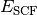.

(4)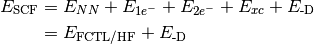Unless the method includes a dispersion correction, this quantity is equal to HF TOTAL ENERGY (for HF) or DFT FUNCTIONAL TOTAL ENERGY (for DFT). Unless the method is a DFT double-hybrid, this quantity is equal to DFT TOTAL ENERGY.

TWO-ELECTRON ENERGY

The two-electron energy contribution [H] to the total SCF energy. Quantity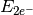in Eq. (4).

UNCP-CORRECTED 2-BODY INTERACTION ENERGY

The interaction energy [H] considering only two-body interactions, computed without counterpoise correction. Related variable CP-CORRECTED 2-BODY INTERACTION ENERGY.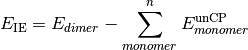ZAPTn TOTAL ENERGY
ZAPTn CORRELATION ENERGY

The total electronic energy [H] and correlation energy component [H] for the labeled Z-averaged perturbation theory level. n is ZAPT perturbation order.